Like   Tweet   in

## REPORT YOUR RISKS IN CONFLUENCE WITH THE BRAND NEW SOFTCOMPLY RISK MANAGER EXTENSION!

 table div table+table+table div table,table.module-2{width:27.74%;float:left;padding:0}table div table+table+table div table a{border:0 none;text-decoration:none}table div table+table+table div table img{width:100%!important;border:0 none;text-decoration:none}table div table+table+table div table td{width:100%;padding:0 20px 20px 0}/* styles */ Managing risks in Jira is great - you can use the flexibility of Jira to add various fields to the risk table, to customise the table and the matrices and filter/sort the data any which way you want. But what if you'd like to report your risks in Confluence? That is especially important for all of those companies that may want to add risk matrices and risk table views to product risk reports, the templates for which you may already have created within Confluence. We are happy to inform you that we have just released an extension to the SoftComply Risk Manager app on Jira Cloud -> SoftComply Risk Manager for Confluence! This is a free extension that displays Risk Table and Risk Matrix macros on your Confluence pages. To check out all the features of the app, please see the User Guide of the SoftComply Risk Manager for Confluence. Below is a short step-by-step guide on how to get started with the new app.
 table div table+table+table+table div table{width:100%;padding:0}table div table+table+table+table div table img{width:96.23%;padding:0;float:none}table div table+table+table+table div table td{width:100%;padding:0 1.88% 18px}/* styles */## Step 1 - add a Risk Macro on your Confluence page

 /* styles */ After installing the free Confluence app, simply add a macro onto your Confluence page of either Risk Matrix or Risk Table, depending on the information you wish to display.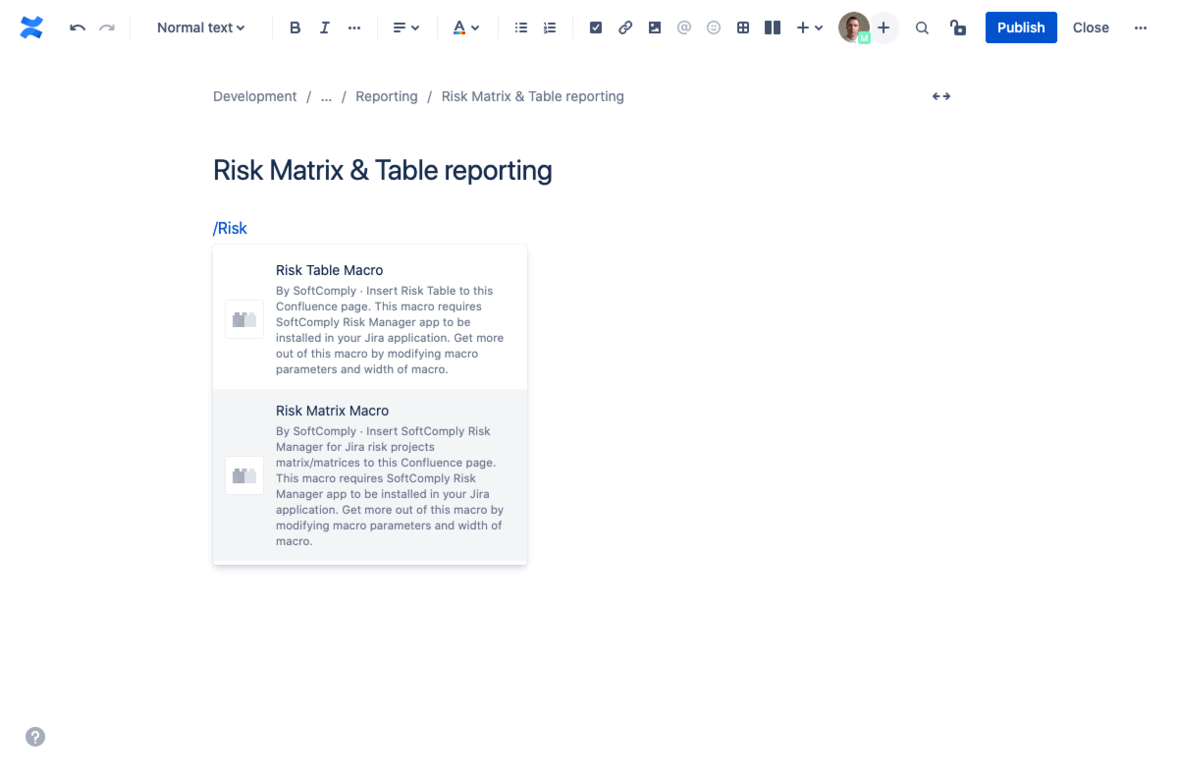table div table+table+table+table+table+table+table+table div table{width:100%;padding:0}table div table+table+table+table+table+table+table+table div table img{width:96.23%;padding:0;float:none}table div table+table+table+table+table+table+table+table div table td{width:100%;padding:0 1.88% 18px}/* styles */## Step 2 - Configure the Macro

 /* styles */ To customise the risk information to be displayed, go to the Macro Settings to specify what and how you would like to see the information on the page. For the Risk Matrix macro, you can choose the risk project from where the information is displayed, the matrices you would like to show (either Initial, Residual or both) and additional information about the risks like Risk ID numbers, names and descriptions of Risk Classes, Severity and Probability Levels.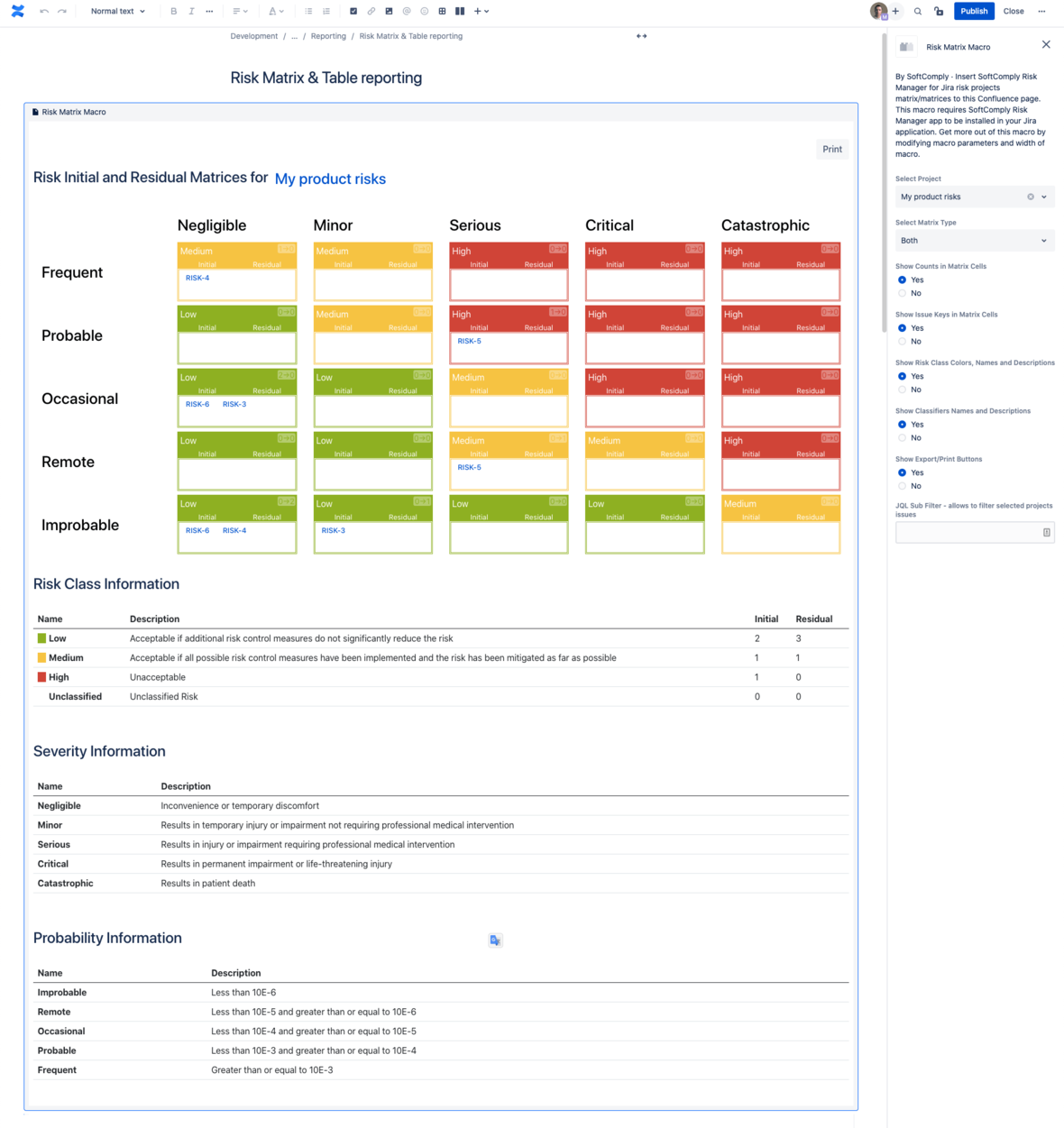/* styles */ For the Risk Table macro, you can choose the risk project from where the information is displayed, the number of risks per page and in case you would like to display only a set of risks, the exact JQL Filter for it.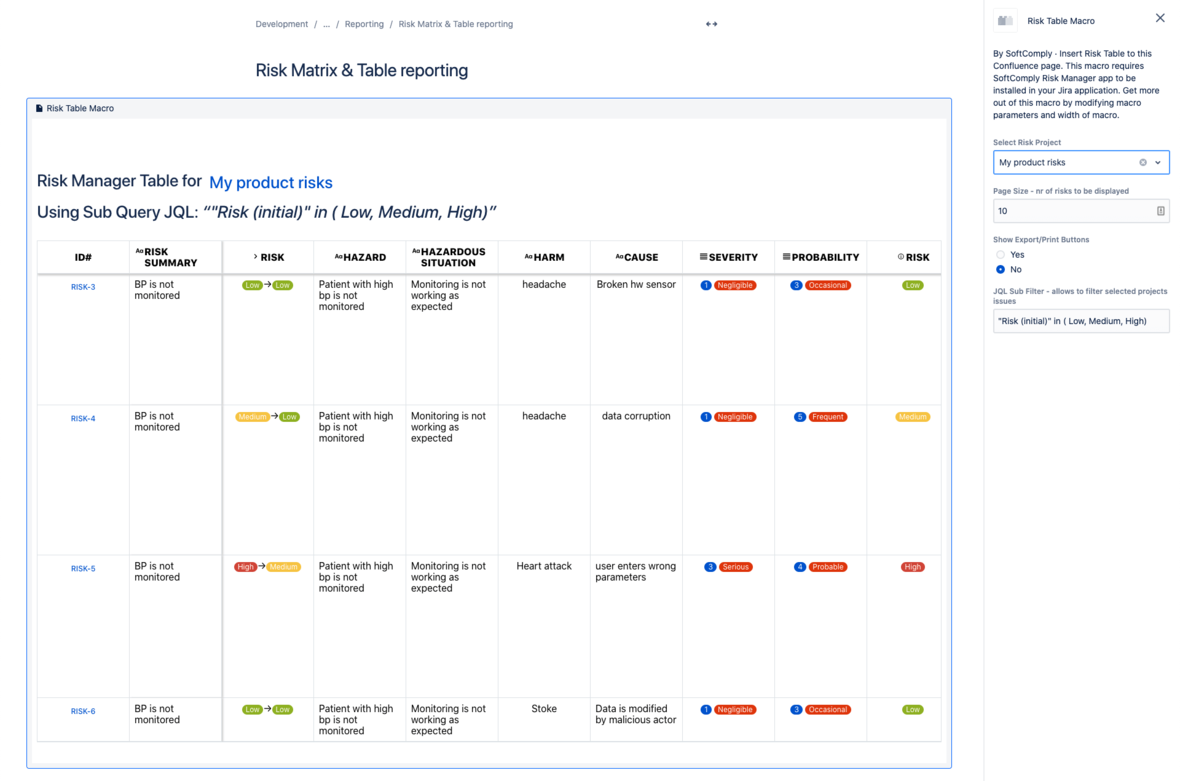table div table+table+table+table+table+table+table+table+table+table+table+table+table+table div table{width:100%;padding:0}table div table+table+table+table+table+table+table+table+table+table+table+table+table+table div table img{width:96.23%;padding:0;float:none}table div table+table+table+table+table+table+table+table+table+table+table+table+table+table div table td{width:100%;padding:0 1.88% 18px}/* styles */## Step 3 - Customize your Risk Report on your Confluence Page

 /* styles */ You can now have your Risk Matrices displayed on your Confluence page with all the details about the Risk Classes, Severity and Probability Levels. You can further customise the page for any type of report that you need.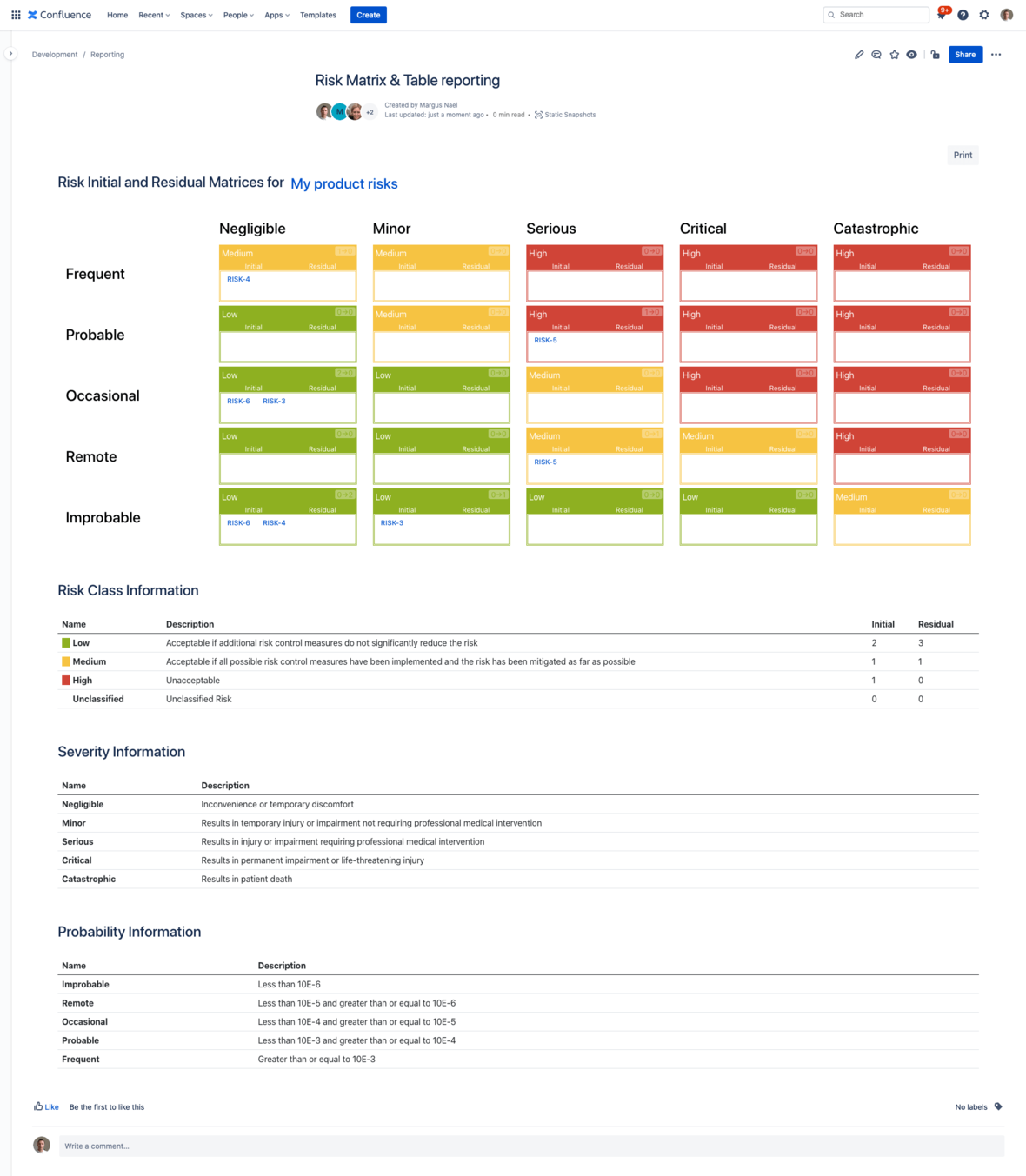table div table+table+table+table+table+table+table+table+table+table+table+table+table+table+table+table+table+table div table{width:100%;padding:0}table div table+table+table+table+table+table+table+table+table+table+table+table+table+table+table+table+table+table div table img{width:96.23%;padding:0;float:none}table div table+table+table+table+table+table+table+table+table+table+table+table+table+table+table+table+table+table div table td{width:100%;padding:0 1.88% 18px}/* styles *//* styles */ Now that you have your Risk Table on your Confluence page from the risk project of your choice, you can write necessary text around it for reporting as needed. To be able to easily navigate in the table, the Risk ID and Summary columns have been frozen when scrolling to the right.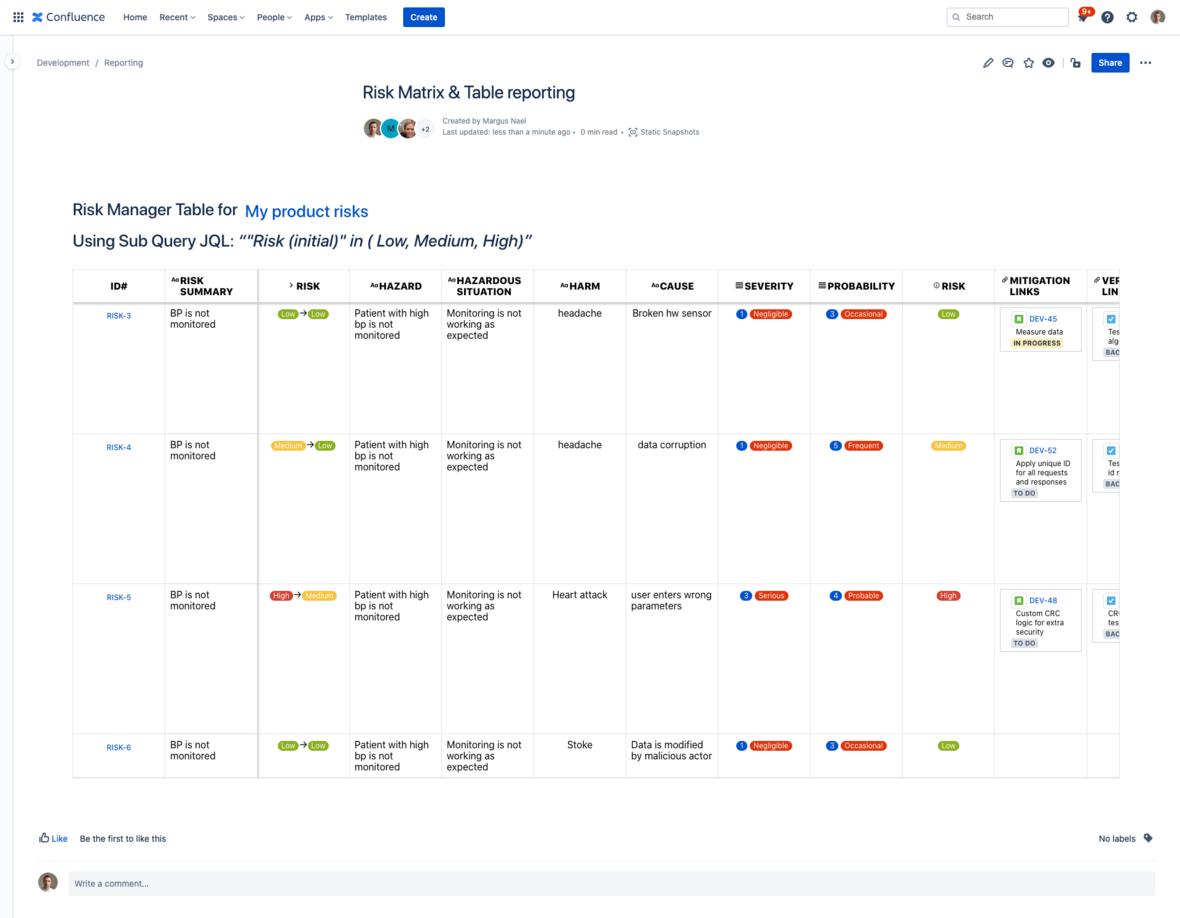table div table+table+table+table+table+table+table+table+table+table+table+table+table+table+table+table+table+table+table+table+table div table{width:100%;padding:0}table div table+table+table+table+table+table+table+table+table+table+table+table+table+table+table+table+table+table+table+table+table div table img{width:96.23%;padding:0;float:none}table div table+table+table+table+table+table+table+table+table+table+table+table+table+table+table+table+table+table+table+table+table div table td{width:100%;padding:0 1.88% 18px}/* styles */## Check out the Short Video Tutorial:

 table.module-22{width:75.85%;padding:0}table div table+table+table+table+table+table+table+table+table+table+table+table+table+table+table+table+table+table+table+table+table+table+table div table{width:75.85%;float:none;margin-left:auto;margin-right:auto;padding:0}table div table+table+table+table+table+table+table+table+table+table+table+table+table+table+table+table+table+table+table+table+table+table+table div table a{border:0 none;text-decoration:none}table div table+table+table+table+table+table+table+table+table+table+table+table+table+table+table+table+table+table+table+table+table+table+table div table img{width:100%!important;border:0 none;text-decoration:none}table div table+table+table+table+table+table+table+table+table+table+table+table+table+table+table+table+table+table+table+table+table+table+table div table td{width:100%;padding:0}/* styles */
 table div table+table+table+table+table+table+table+table+table+table+table+table+table+table+table+table+table+table+table+table+table+table+table+table div table{width:100%;padding:0}table div table+table+table+table+table+table+table+table+table+table+table+table+table+table+table+table+table+table+table+table+table+table+table+table div table img{width:96.23%;padding:0;float:none}table div table+table+table+table+table+table+table+table+table+table+table+table+table+table+table+table+table+table+table+table+table+table+table+table div table td{width:100%;padding:0 1.88% 18px}/* styles */## Try it out from Atlassian Marketplace!

 table.module-25{width:75.47%;padding:0}table div table+table+table+table+table+table+table+table+table+table+table+table+table+table+table+table+table+table+table+table+table+table+table+table+table+table div table{width:75.47%;float:none;margin-left:auto;margin-right:auto;padding:0}table div table+table+table+table+table+table+table+table+table+table+table+table+table+table+table+table+table+table+table+table+table+table+table+table+table+table div table a{border:0 none;text-decoration:none}table div table+table+table+table+table+table+table+table+table+table+table+table+table+table+table+table+table+table+table+table+table+table+table+table+table+table div table img{width:100%!important;border:0 none;text-decoration:none}table div table+table+table+table+table+table+table+table+table+table+table+table+table+table+table+table+table+table+table+table+table+table+table+table+table+table div table td{width:100%;padding:0}/* styles */
 table div table+table+table+table+table+table+table+table+table+table+table+table+table+table+table+table+table+table+table+table+table+table+table+table+table+table+table div table{width:100%;padding:0}table div table+table+table+table+table+table+table+table+table+table+table+table+table+table+table+table+table+table+table+table+table+table+table+table+table+table+table div table img{width:96.23%;padding:0;float:none}table div table+table+table+table+table+table+table+table+table+table+table+table+table+table+table+table+table+table+table+table+table+table+table+table+table+table+table div table td{width:100%;padding:0 1.88% 18px}/* styles */## In case of questions or to book a demo call, please let us know!

 table.module-28{width:75.47%;padding:0}table div table+table+table+table+table+table+table+table+table+table+table+table+table+table+table+table+table+table+table+table+table+table+table+table+table+table+table+table+table div table{width:75.47%;float:none;margin-left:auto;margin-right:auto;padding:0}table div table+table+table+table+table+table+table+table+table+table+table+table+table+table+table+table+table+table+table+table+table+table+table+table+table+table+table+table+table div table a{border:0 none;text-decoration:none}table div table+table+table+table+table+table+table+table+table+table+table+table+table+table+table+table+table+table+table+table+table+table+table+table+table+table+table+table+table div table img{width:100%!important;border:0 none;text-decoration:none}table div table+table+table+table+table+table+table+table+table+table+table+table+table+table+table+table+table+table+table+table+table+table+table+table+table+table+table+table+table div table td{width:100%;padding:0}/* styles */